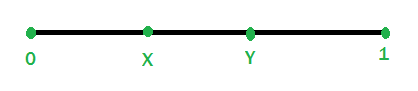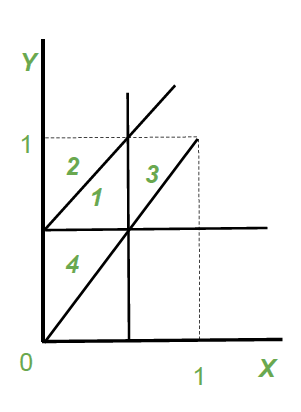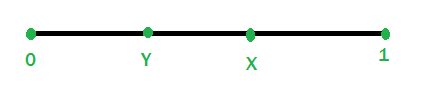Probability of cutting a rope into three pieces such that the sides form a triangle

• Difficulty Level : Medium
• Last Updated : 29 Aug, 2018

Given a rope. We have to find the probability of cutting a rope into 3 pieces such that they form a triangle.

Explanation:
Let the length of rope be 1 unit. We choose two points X and Y on the rope.

Note: Formation of triangle is based on Triangle inequality i.e. sum of the lengths of any two sides of a triangle must be greater than the length of the third side

There are two possibilities of choosing points X and Y on rope:
Case 1: X < Y

Length of pieces after choosing points X and Y:
X units, (Y-X) units, (1-Y) units
Below line diagram shows the partition rope.The 3 possible combination for satisfying Triangle Inequality

1. X + (Y-X) > (1-Y)
=> 2Y > 1
=> Y > (1/2)

2. X + (1-Y) > (Y-X)
=> 2X + 1 > 2Y
=> Y < X + (1/2)

3. (Y-X) + (1-Y) > X
=> 2X < 1
=> X < 1/2

Forming a graph using above 3 conditions and X<YThe area in the region 1 of the graph is the required area which is: 1/2*1/2*1/2 = 1/8

Case 2: X > Y

Length of pieces after choosing points X and Y:
Y units, (X-Y) units, (1-X) units
Below line diagram shows the partition rope.The 3 possible combinations for satisfying Triangle Inequality

1. Y + (X-Y) > (1-X)
=> 2X > 1
=> X > (1/2)

2. Y + (1-X) > (X-Y)
=> 2Y + 1 > 2X
=> X < Y + (1/2)

3. (X-Y) + (1-X) > Y
=> 2Y < 1
=> Y < 1/2

Forming a Graph using 3 condition and Y<XThe area in the region 5 of the graph is the required area which is: 1/2*1/2*1/2 = 1/8

In both cases we get same required area, thus area required will be: 1/8.
So the Probability of breaking a rope into three pieces such that the sides form a triangle
is given by:
Area required/Total area = (1/8)/(1/2*1*1) = 1/4 = 0.25.

My Personal Notes arrow_drop_up